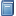Buchempfehlung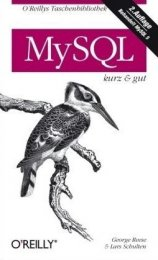MySQL kurz & gut
Das preiswerte Taschen- buch stellt MySQL-rele- vante Inhalte systematisch und knapp dar, sodass es sich optimal zum Nach- schlagen beim Pro- grammieren eignet. [Mehr Infos...]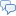FreeBASIC-Chat
Es sind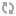Benutzer im FreeBASIC-Chat online.
(Stand:  )

# fb:porticula NoPaste

## hiddendata.bas

 Uploader: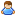ThePuppetMaster Datum/Zeit: 20.06.2009 01:07:04
Hinweis: Dieser Quelltext ist Bestandteil des Projekts HiddenData, zu dem es auf FreeBASIC-Portal.de eine Projektseite gibt.

```'###################################################################################### '<APP_Data><Hidden_Data><4Byte_HiddenDataLen><4Byte_HiddenDataAllocLen><4Byte_AppLen> '###################################################################################### Function HiddenData_Get() as String Dim XName as String = Command(0) #If Defined(__fb_linux__)     XName = Mid(XName, InStrRev(XName, "/") + 1) #ElseIf Defined(__fb_win32__)     XName = Mid(XName, InStrRev(XName, "\") + 1) #EndIf Dim XFN1 as Integer = FreeFile If Open(XName For Binary as #XFN1) <> 0 Then Return "" Dim XLen as UInteger = Lof(XFN1) Dim D as String = Space(12) Get #XFN1, XLen - 11, D Dim XALen as UInteger = (D shl 24) or (D shl 16) or (D shl 8) or D Dim XLLen as UInteger = (D shl 24) or (D shl 16) or (D shl 8) or D Dim XDLen as UInteger = (D shl 24) or (D shl 16) or (D shl 8) or D If XALen = 0 Then Close #XFN1: Return "" If XLLen = 0 Then Close #XFN1: Return "" If XDLen = 0 Then Close #XFN1: Return "" If XLen <> (XALen + XLLen + 12) Then Close #XFN1: Return "" D = Space(XDLen) Get #XFN1, XALen + 1, D Close #XFN1 Return D End Function '###################################################################################### Sub HiddenData_Del() Dim XName as String = Command(0) #If Defined(__fb_linux__)     XName = Mid(XName, InStrRev(XName, "/") + 1) #ElseIf Defined(__fb_win32__)     XName = Mid(XName, InStrRev(XName, "\") + 1) #EndIf Dim XFN2 as Integer = FreeFile If Open(XName For Binary as #XFN2) <> 0 Then Exit Sub Dim XFN1 as Integer = FreeFile If Open(XName & " " For Binary as #XFN1) <> 0 Then Close #XFN2: Exit Sub Dim XLen as UInteger = Lof(XFN2) Dim D as String = Space(6000) For X as UInteger = 1 to XLen Step 6000     If XLen - X < 6000 Then D = Space(XLen - X + 1)     Get #XFN2, X, D     Put #XFN1, X, D Next Close #XFN2 D = Space(12) Get #XFN1, XLen - 11, D Dim XALen as UInteger = (D shl 24) or (D shl 16) or (D shl 8) or D Dim XLLen as UInteger = (D shl 24) or (D shl 16) or (D shl 8) or D Dim XDLen as UInteger = (D shl 24) or (D shl 16) or (D shl 8) or D If XALen = 0 Then Close #XFN1: Exit Sub If XLLen = 0 Then Close #XFN1: Exit Sub If XDLen = 0 Then Close #XFN1: Exit Sub If XLen <> (XALen + XLLen + 12) Then Close #XFN1: Exit Sub Put #XFN1, XLen - (XDLen + 12), String(XDLen + 12, 0) Close #XFN1 #If Defined(__fb_linux__)     XFN1 = FreeFile     Open Pipe "ls -l " & XName for input as #XFN1     Do Until Eof(XFN1)         Line Input #XFN1, D         D = Left(D, 10)         Exit Do     Loop     Close #XFN1 #EndIf Kill XName Name XName & " " as XName #If Defined(__fb_linux__)     If Right(D, 1) = "x" Then         XFN1 = FreeFile         Open Pipe "chmod +x " & XName for input as #XFN1         Do Until Eof(XFN1)             Exit Do         Loop         Close #XFN1     End If #EndIf End Sub '###################################################################################### Sub HiddenData_Set(V_Data as String) HiddenData_Del() Dim XName as String = Command(0) #If Defined(__fb_linux__)     XName = Mid(XName, InStrRev(XName, "/") + 1) #ElseIf Defined(__fb_win32__)     XName = Mid(XName, InStrRev(XName, "\") + 1) #EndIf Dim XFN2 as Integer = FreeFile If Open(XName For Binary as #XFN2) <> 0 Then Exit Sub Dim XFN1 as Integer = FreeFile If Open(XName & " " For Binary as #XFN1) <> 0 Then Close #XFN2: Exit Sub Dim XLen as UInteger = Lof(XFN2) Dim D as String = Space(6000) For X as UInteger = 1 to XLen Step 6000     If XLen - X < 6000 Then D = Space(XLen - X + 1)     Get #XFN2, X, D     Put #XFN1, X, D Next Close #XFN2 D = Space(12) Get #XFN1, XLen - 11, D Dim XALen as UInteger = (D shl 24) or (D shl 16) or (D shl 8) or D Dim XLLen as UInteger = (D shl 24) or (D shl 16) or (D shl 8) or D Dim XDLen as UInteger = Len(V_Data) If XLLen < XDLen Then XLLen = XDLen Dim XSAL as String = Chr((XALen shr 24) and 255) & Chr((XALen shr 16) and 255) & Chr((XALen shr 8) and 255) & Chr(XALen and 255) Dim XSLL as String = Chr((XLLen shr 24) and 255) & Chr((XLLen shr 16) and 255) & Chr((XLLen shr 8) and 255) & Chr(XLLen and 255) Dim XSDL as String = Chr((XDLen shr 24) and 255) & Chr((XDLen shr 16) and 255) & Chr((XDLen shr 8) and 255) & Chr(XDLen and 255) If XLen <> (XALen + XLLen + 12) Then     XALen = XLen     XLLen = XDLen     XSAL = Chr((XALen shr 24) and 255) & Chr((XALen shr 16) and 255) & Chr((XALen shr 8) and 255) & Chr(XALen and 255)     XSLL = Chr((XLLen shr 24) and 255) & Chr((XLLen shr 16) and 255) & Chr((XLLen shr 8) and 255) & Chr(XLLen and 255)     Put #XFN1, XALen + 1, V_Data & XSDL & XSLL & XSAL Else     Put #XFN1, XALen, V_Data     Put #XFN1, (XALen + XLLen) - 11, XSDL & XSLL & XSAL End If Close #XFN1 #If Defined(__fb_linux__)     XFN1 = FreeFile     Open Pipe "ls -l " & XName for input as #XFN1     Do Until Eof(XFN1)         Line Input #XFN1, D         D = Left(D, 10)         Exit Do     Loop     Close #XFN1 #EndIf Kill XName Name XName & " " as XName #If Defined(__fb_linux__)     If Right(D, 1) = "x" Then         XFN1 = FreeFile         Open Pipe "chmod +x " & XName for input as #XFN1         Do Until Eof(XFN1)             Exit Do         Loop         Close #XFN1     End If #EndIf End Sub '###################################################################################### Dim D as String D = HiddenData_Get() Print "GET_Len:  "; Str(Len(D)) Print "GET_Data:>"; D; "<" Print HiddenData_Set("Test") Print "SET:     >Test<" Print D = HiddenData_Get() Print "GET_Len:  "; Str(Len(D)) Print "GET_Data:>"; D; "<" Print HiddenData_Del() Print "DEL" Print D = HiddenData_Get() Print "GET_Len:  "; Str(Len(D)) Print "GET_Data:>"; D; "<" Print HiddenData_Set("1234") Print "SET:     >1234<" Print D = HiddenData_Get() Print "GET_Len:  "; Str(Len(D)) Print "GET_Data:>"; D; "<" Print End 0```